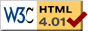### Journée-séminaire de combinatoire

#### (équipe CALIN du LIPN, université Paris-Nord, Villetaneuse)

Le 23 novembre 2021 à 14h00 en B107 (& visioconférence), Lionel Pournin nous parlera de : Primitive point packing: number theory and geometric combinatorics

Résumé : A point from the grid ${\mathbb Z}^d$ is called primitive when its coordinates are relatively prime. In this talk we will examine the question of how many primitive points one can pick, whose first non-zero coordinate is positive, under the constraint that the largest coordinate of their sum is at most a fixed number $k$. We will solve the question exactly for all $k$ and $d$. A number of connections with number theory, geometric combinatorics, and the theory of linear programming will be made explicit along the way.

 Dernière modification : Monday 24 January 2022Contact pour cette page : Cyril.Banderier at lipn.univ-paris13.fr